# AB Calculus Test Practice Questions with Answers Sample 1

A set of AB calculus questions, with answers, similar to the questions in the AP calculus test are presented. The answers to the suggested questions are at the bottom of the page. Detailed solutions and explanations are also included.= A) e B) 1 C) eh D) e4 E) 4e The graph of function g defined by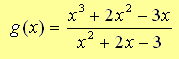will have vertical asymptotes at A)x = 1 , -3 B) x = 0 C) x = 1 D) x = -3 E) Function g has no vertical asymptotes Given thatfindA) 2/3 B) 4/3 C) 1/3 D) 2 E) Does not exist Function f is defined by. Find df(x) / dx. A) 6x2 sin(x) - (1/x2)tan(x) + sec(x) B) 6x2 sin(x) + 2x3 cos(x) - (1/x2)tan(x) + (1/x) sec2(x)) + sec(x) + x sin(x) sec2(x) C) 2x3 cos(x) + 1/x sec2(x)) + x sin(x) sec2(x) D) 6x2 cos(x) - (1/x2 sec2(x)) + sec2(x) E) 6x2 sin(x) + 2x3 cos(x) - (1/x2)tan(x) + (1/x sec2(x)) + sec(x) + x sin(x) sec2(x) + 2 Curve C is described by the equation 0.25x2 + y2 = 9. Determine the y coordinates of the points on curve C whose tangent lines have slope equal to 1. A) -3 sqrt(5) / 5 , 3 sqrt(5) / 5 B) - sqrt(35) / 2 , sqrt(35) / 2 C) -3 , 3 D) - sqrt(2) / 2 , sqrt(2) / 2 E) -3 sqrt(2) , 3 sqrt(2) Find the solution to the differential equation dy/dx = cos(x) / y2 , where y(π/2) = 0. A) y = (3 sin(x) - 3) B) y = sin(x) - 1 C) y = (3 sin(x) - 3)1/3 D) y = (3 sin(x) - 3)3 E) y = (3 sin(x) - 3)-1/3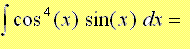A) cos5(x) + C B) -(1/5)sin5(x) + C C) sin5(x) + C D) -(1/5)cos5(x) + C E) -5cos5(x) + CA) 2sin(4x2 + 1) B) 2sin(x2 + 1) C) sin(x2 + 1) D) 2 sin(4x2 + 1) - 2 sin(32 + 1) E) 2 sin(4x2)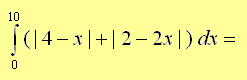A) 100 B) 108 C) 110 D) 112 E) 114 Evaluate the integralA) (5 + x3/4)10 B) (x3/4)10 C) (1/10)(5 + x3/4)10 D) (1/10)(5 + x3/4)10 / x1/4 E) (2/15)(5 + x3/4)10 Given that function h is defined byfind h'(x). A) (3x2 / (x6 + 2x3 + 2) + 2) B) 4 (arctan(x3 + 1) + 2x)3 (3x2 / (x6 + 2x3 + 2) ) C) 4 (arctan(x3 + 1) + 2x)3 D) 4 (3x2 / (x6 + 2x3 + 2) + 2) E) (1/4)(arctan(x3 + 1) + 2x)3 The graph of function h is shown below. How many zeros does the first derivative h' of h have?A) 1 B) 2 C) 3 D) 4 E) 5 The graph of a polynomial f is shown below. If f' is the first derivative of f, then the remainder of the division of f'(x) by x - b is more likely to be equal toA) f(b) B) 1 C) 0 D) 2 E) -1 The set of all points (ln(t - 2) , 3t), where t is a real number greater than 2, is the graph of A) y = ln(x/3 - 2) B) y = 3x C) x = ln(y - 2) D) y = 3(ex + 2) E) y = ln(x) Let P(x) = 2 x3 + K x + 1. Find K if the remainder of the division of P(x) by x - 2 is equal to 10. A) -7/2 B) 2/7 C) 7/2 D) -2/7 E) K cannot be determined Function f is defined by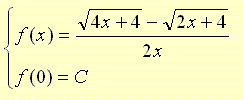. where C is a constant. What must the value of C be equal to for function f to be continuous at x = 0? A) 0 B) 1/4 C) 1/8 D) 1 E) Any real number f and g are functions such that f'(x) = g(x) and g'(x) = f(x). The second derivative of (f . g)(x) is equal to A) f"(x) g"(x) B) g'(x) g(x) + f(x) f'(x) C) 4 g(x) f(x) D) 2 g(x) f(x) E) g(x) f(x) The average rate of change of the function f defined by f(x) = sin(x) + x on the closed interval [0 , pi] is equal to A) 0 B) 2 pi C) pi D) 2 E) 1 The figure shows the graphs of y = sin(x) over half a period and the line y = 1/2. Find area of the shaded region.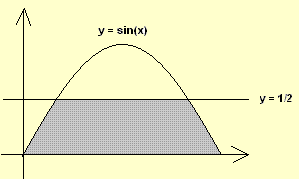. A) 1 B) 0.5 C) 2 + π/3 D) 2 + π/3 - √3 E) 2 + π/3 + √3 Functions f, g and h are defined as follow: g(x) = f(x2), f(x) = h(x3 + 1) and h'(x) = 2x + 1. g'(x) = A) 2 x3 B) 12 x9 + 18 x3 C) 2 x11 + 3 x5 D) 2 x9 + 3 x3 E) 12 x11 + 18 x5 Answers to the Above Questions D E A B A C D A B E B E C D A B C E D E